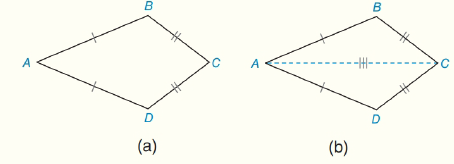Chapter 4.CT, Problem 15CT### Elementary Geometry for College St...

6th Edition
Daniel C. Alexander + 1 other
ISBN: 9781285195698

#### Solutions

Chapter
Section### Elementary Geometry for College St...

6th Edition
Daniel C. Alexander + 1 other
ISBN: 9781285195698
Textbook Problem
64 views

# Complete the proof of the following theorem:“In a kite, one pair of opposite angles are congruent.”Given: Kite ABCD; A B ¯ ≅ A D ¯   and  B C ¯ ≅ D C ¯ Prove: ∠ B ≅ ∠ DPROOF Statements Reasons 1_________2. Draw A C ¯ 3.__________4. Δ A C D ≅ Δ A C B 5.__________ 1.______________2. Through two points, there is exactly one line.3. Identity4.____________5._________________

To determine

To Find:

The proof of BD

Explanation

Given:

Kite ABCD,

Approach:

Consider the theorem,

“In a kite, one pair of opposite angles are congruent.”

 PROOF Statements Reasons 1. AB¯≅AD¯ and BC¯≅DC¯2. Draw AC¯3. AC¯≅AC¯4. ΔACD≅ΔACB5

### Still sussing out bartleby?

Check out a sample textbook solution.

See a sample solution

#### The Solution to Your Study Problems

Bartleby provides explanations to thousands of textbook problems written by our experts, many with advanced degrees!

Get Started